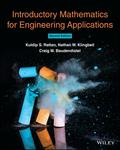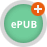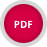John Wiley & Sons Introductory Mathematics for Engineering ApplicationsIntroductory Mathematics for Engineering Applications, 2nd Edition, provides first-year engineering .. Product #: 978-1-119-60442-6 Regular price: \$69.07 \$69.07 In Stock

# Introductory Mathematics for Engineering Applications2. Edition July 2021
464 Pages, Softcover
Wiley & Sons Ltd

ISBN: 978-1-119-60442-6
John Wiley & Sons

### Price: 73,90 €

Price incl. VAT, excl. Shipping

Further versionsIntroductory Mathematics for Engineering Applications, 2nd Edition, provides first-year engineering students with a practical, applications-based approach to the subject. This comprehensive textbook covers pre-calculus, trigonometry, calculus, and differential equations in the context of various discipline-specific engineering applications. The text offers numerous worked examples and problems representing a wide range of real-world uses, from determining hydrostatic pressure on a retaining wall to measuring current, voltage, and energy stored in an electrical capacitor.

Rather than focusing on derivations and theory, clear and accessible chapters deliver the hands-on mathematical knowledge necessary to solve the engineering problems students will encounter in their careers. The textbook is designed for courses that complement traditional math prerequisites for introductory engineering courses -- enabling students to advance in their engineering curriculum without first completing calculus requirements. Now available in enhanced ePub format, this fully updated second edition helps students apply mathematics to engineering scenarios involving physics, statics, dynamics, strength of materials, electric circuits, and more.

PREFACE vii

ACKNOWLEDGEMENT ix

1 STRAIGHT LINES IN ENGINEERING 1

1.1 Vehicle during Braking 1

1.2 Voltage-Current Relationship in a Resistive Circuit 3

1.3 Force-Displacement in a Preloaded Tension Spring 6

1.4 Further Examples of Lines in Engineering 7

Problems 18

2 QUADRATIC EQUATIONS IN ENGINEERING 31

2.1 A Projectile in a Vertical Plane 31

2.2 Current in a Lamp 35

2.3 Equivalent Resistance 36

2.4 Further Examples of Quadratic Equations in Engineering 38

Problems 50

3 TRIGONOMETRY IN ENGINEERING 61

3.1 Introduction 61

3.2.1 Kinematics of One-Link Robot 61

3.2.2 Inverse Kinematics of One-Link Robot 69

3.3.1 Direct Kinematics of Two-Link Robot 74

3.3.2 Inverse Kinematics of Two-Link Robot 76

3.3.3 Further Examples of Two-Link Planar Robot 81

3.4 Further Examples of Trigonometry

in Engineering 91

Problems 99

4 TWO-DIMENSIONAL VECTORS IN ENGINEERING 107

4.1 Introduction 107

4.2 Position Vector in Rectangular Form 108

4.3 Position Vector in Polar Form 108

4.4.1 Examples of Vector Addition in Engineering 112

Problems 124

5 COMPLEX NUMBERS IN ENGINEERING 134

5.1 Introduction 134

5.2 Position of One-Link Robot as

a Complex Number 135

5.3 Impedance of R, L, and C as a Complex Number 136

5.3.1 Impedance of a Resistor R 136

5.3.2 Impedance of an Inductor L 136

5.3.3 Impedance of a Capacitor C 137

5.4 Impedance of a Series RLC Circuit 137

5.5 Impedance of R and L Connected in Parallel 139

5.6 Armature Current in a DC Motor 141

5.7 Further Examples of Complex Numbers in Electric Circuits 143

5.8 Complex Conjugate 148

Problems 149

6 SINUSOIDS IN ENGINEERING 160

6.1 One-Link Planar Robot as a Sinusoid 160

6.2 Angular Motion of the One-Link Planar Robot 163

6.2.1 Relations between Frequency and Period 164

6.3 Phase Angle, Phase Shift, and Time Shift 165

6.4 General Form of a Sinusoid 167

6.5 Addition of Sinusoids of the Same Frequency 169

Problems 176

7 SYSTEMS OF EQUATIONS IN ENGINEERING 188

7.1 Introduction 188

7.2 Solution of a Two-Loop Circuit 188

7.3 Tension in Cables 193

7.4 Further Examples of Systems of Equations in Engineering 196

Problems 210

8 DERIVATIVES IN ENGINEERING 223

8.1 Introduction 223

8.1.1 What Is a Derivative? 223

8.2 Maxima and Minima 226

8.3 Applications of Derivatives in Dynamics 230

8.3.1 Position, Velocity, and Acceleration 230

8.4 Applications of Derivatives in Electric Circuits 245

8.4.1 Current and Voltage in an Inductor 248

8.4.2 Current and Voltage in a Capacitor 252

8.5 Applications of Derivatives in Strength of Materials 255

8.6 Further Examples of Derivatives in Engineering 265

Problems 270

9 INTEGRALS IN ENGINEERING 282

9.1 Introduction: The Asphalt Problem 282

9.2 Concept of Work 287

9.3 Application of Integrals in Statics 290

9.3.1 Center of Gravity (Centroid) 290

9.3.2 Alternate Definition of the Centroid 298

9.4.1 Hydrostatic Pressure on a Retaining Wall 301

9.5 Applications of Integrals in Dynamics 307

9.5.1 Graphical Interpretation 313

9.6 Applications of Integrals in Electric Circuits 319

9.6.1 Current, Voltage, and Energy Stored in a Capacitor 319

9.7 Current and Voltage in an Inductor 328

9.8 Further Examples of Integrals in Engineering 333

Problems 342

10 DIFFERENTIAL EQUATIONS IN ENGINEERING 354

10.1 Introduction: The Leaking Bucket 354

10.2 Differential Equations 355

10.3 Solution of Linear DEQ with Constant Coefficients 356

10.4 First-Order Differential Equations 357

10.5 Second-Order Differential Equations 383

10.5.1 Free Vibration of a Spring-Mass System 383

10.5.2 Forced Vibration of a Spring-Mass System 387

10.5.3 Second-Order LC Circuit 394

Problems 397

11 PROBABILITY AND STATISTICS IN ENGINEERING 409

11.1 Introduction 409

11.2 Quality Control Probability in Manufacturing 409

11.3 Manufacturing Tolerance of Resistors 412

11.4 Probability of Accepting/Rejecting

Manufactured Resistors 414

Problems 420